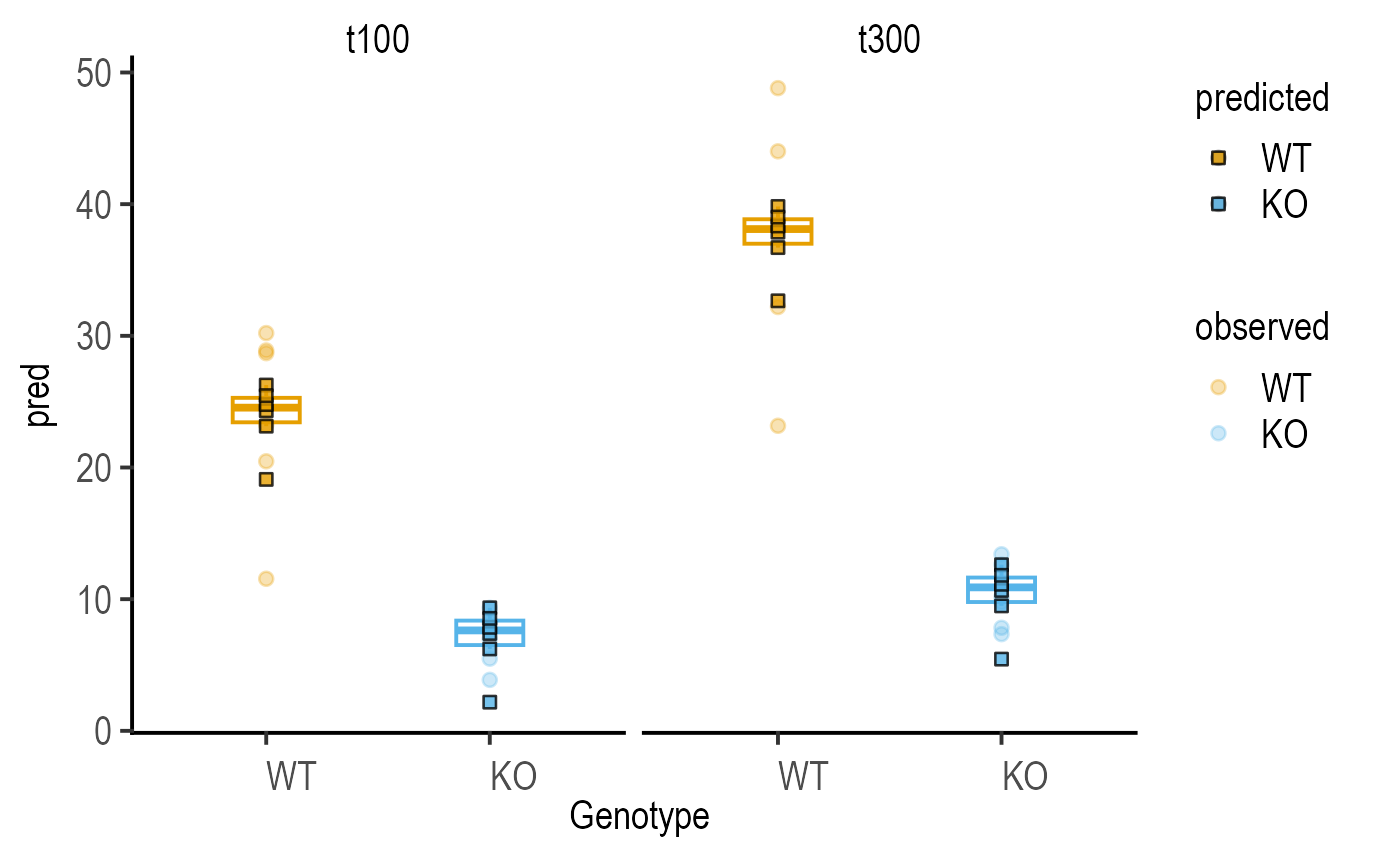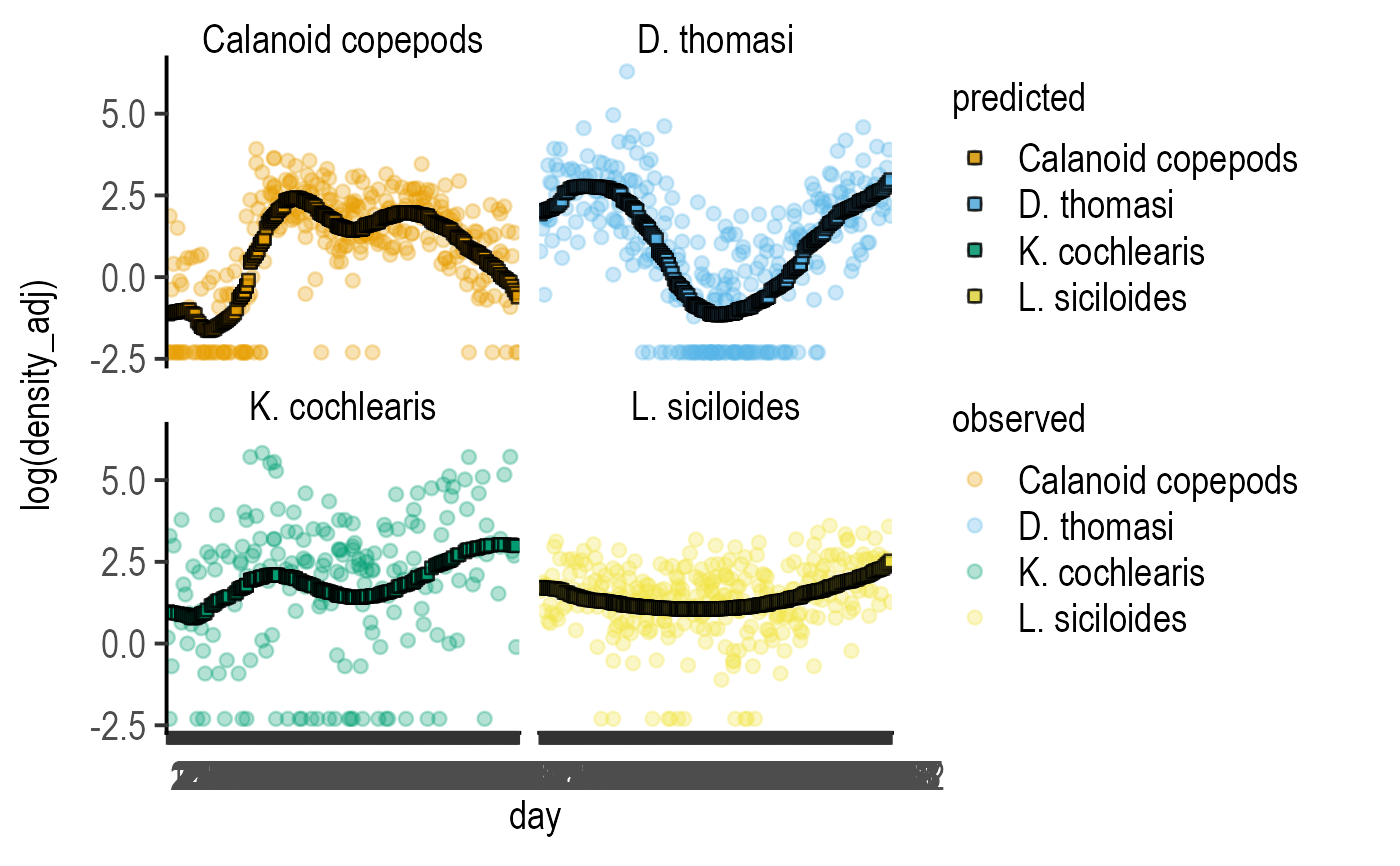This function takes a linear model, and up to three variables and plots observe data (circles) and model predictions (squares). If the X-variable is categorical, a box and whiskers plot is overlaid. A variable (ByFactor) can be used for faceting.

plot_lm_predict(
Model,
xcol,
ycol,
ByFactor,
obs_size = 2,
obs_alpha = 0.3,
pred_size = 2,
pred_alpha = 0.8,
linethick,
base_size = 15,
...
)

## Arguments

Model

a linear model saved with simple_model, mixed_model or ga_model.

xcol

variable along the X axis (should match one of the dependent variables in model exactly).

ycol

independent variable along the Y axis (should match independent variable in model exactly).

ByFactor

optional faceting variable (should match one of the variables in model exactly).

obs_size

size of symbols for observed data (default = 2).

obs_alpha

opacity of symbols for observed data (default = 0.3).

pred_size

size of symbols for predicted data (default = 2).

pred_alpha

opacity of symbols for predicted data (default = 0.8).

linethick

thickness of border lines for boxes and symbols (default is base_size/20).

base_size

base fontsize for theme_grafify

...

any other parameters to be passed to theme_grafify

## Value

This function returns a ggplot2 object of class "gg" and "ggplot".

## Examples

#fit a model
deathm1 <- mixed_model(data_2w_Tdeath,
"PI", c("Genotype", "Time"),
"Experiment")
#plot model
plot_lm_predict(deathm1,
Genotype, PI, Time)#fit zooplankton data
z1 <- ga_model(data = data_zooplankton,
ycol = log(density_adj),